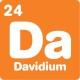# Blog

## Two eggs brainteaser

By

,

Difficulty: Taxing

Jenny found a strange sum written on a piece of paper:
EGG + EGG = PAGE

Each letter represents a different digit, so P might be 1, for example. Can you work out what the sum really is?

Scroll down or click for a hint, or the answer!## Brainteaser hint 1

Writing it upright might make this easier:

EGG
+EGG
PAGE

Look at the ones column and the tens column. Do you see a difference? This could narrow down your options to solve the problem by using algebra, or trial and error.

## Brainteaser hint 2

If you look at just the ones column and the tens column, you’ll see:
GG + GG = GE
Adding GGs together gives two different letters. There must be a 1 being carried!

## Brainteaser hint 3

Still stuck? If carrying a 1, G must be one of these options: 5, 6, 7, 8 or 9.
What answers do you get if you calculate each of these options for GG + GG? What options does that leave for E?If you look at the last two digits of each number, you’ll see:
GG + GG = GE
As mentioned in the hints, there must be a 1 being carried!

Looking at just the tens column:
G + G + 1 = 10 + G
G + G = 9 + G
So, G = 9

Then looking at the ones column:
G + G = 10 + E
9 + 9 = 10 + E
So, E = 8

The final sum is:
899 + 899 = 1798
This reveals the final letters:
P = 1 and A = 7

For more brainteasers and puzzles for kids, subscribe to Double Helix magazine!Categories:

## 3 responses

1.Peter

Seems like a hard way of figuring out out.
Doubling GG produces GE. Trial and error 9 is the only digit that a double of can be doubled to get itself: 99×2=198. And that’s also given us E=8, so from there you can get the final sum.

2.Martin

I went with the trial-and-error method to determine ‘G’

I came to the conclusion that G must be 5 or more because adding G to itself (or doubling it, important later) resulted in two different numbers. The only way that could happen is if there was a carry.

I know that doubling a number always results in an even number. But because of the carry from the Units, we know that G has to be Odd (G+G = even –> plus carry = odd).

Knowing that G is 5 or more and it must be odd, means that it has to be 5, 7, or 9. So, let’s double each and add one (for the carry) and see if the last digit is the same as what we started with. 5+5+1 = 11 (nope) 7+7+1 = 15 (nope) 9+9+1 = 19 (yep)

Once we have “G = 9”, the rest of the equation works itself out.

3.David

Nice solutions you two!

I’m pretty sure I did it with trial and error the first time too. The hints certainly push you down that line of thinking.

I guess I just wanted to see if I could do the algebra!

This site uses Akismet to reduce spam. Learn how your comment data is processed.

By posting a comment you are agreeing to the Double Helix commenting guidelines.